# Important Questions for Class 6 Science Chapter 12 Electricity and Circuits

In this page we have Important Questions for Class 6 Science Chapter 12 Electricity and Circuits . Hope you like them and do not forget to like , social share and comment at the end of the page.
Question 1
What will happen if you join the two terminals of a cell without connecting them through a switch or a bulb?
(a) There will be no impact to cell
(b) The chemicals in the electric cell get used up slowly and cell will produce electricity longer
(c) The chemicals in the electric cell get used up very fast and the cell will no longer produce electric current. It will stop working.
(d) None of these

Question 2
Which is of these are insulators?
(a) Seawater
(b) Distilled water
(c) Tap water
(d) None of these

Question 3
A simple device that is used to either break or complete the electric circuit?
(a) Switch
(b) Terminal
(c) Current
(d) Conductor

Question 4
What is false of electric cell?
(a) Chemical energy is converted into electrical energy
(b) It has a neutral terminal
(c) It has a Positive Terminal
(d) It has a Negative Terminal

Question 5
Which one of the following statement is false?
(a) Eraser is a bad conductor of electricity.
(b) Metals are good conductor of electricity.
(c) Our body is a good conductor of electricity.
(d) Plastic is a good conductor of electricity.

Question 6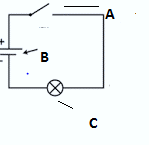(p)A (u) Electric cell (q)B (v)bulb (r)C (w)Switch
(a) P -> W, Q ->U, R-> V
(b) P -> U, Q ->V, R-> W
(c) P -> W, Q ->V, R->U
(d) None of these

Question 7
What is the direction of current flow?
(a) + terminal of cell -> Switch -> Bulb -> - terminal of cell
(b) - terminal of cell -> Switch -> Bulb -> +terminal of cell
(c) + terminal of cell -> - terminal of cell -> bulb -> switch
(d) None of these

Question 8
Materials which allow current to pass through them easily are called?
(a) Insulators
(b) Conductors
(c) Switch
(d) Battery

Question 9
Materials that do not allow electric current to pass through them ?
(a) Insulators
(b) Conductors
(c) Switch
(d) Battery

Question 9
Which one of the following will allow electric current to pass through?
(a) Eraser
(b) Matchstick
(c) Plastic comb
(d) Iron Nail

Question 10
In an electric bulb, light is produced due to
(a) The glass case of the bulb
(b) The gas inside the bulb
(c) the thick wires supporting the filament
(d) the thin filament
Question 11:

Match the column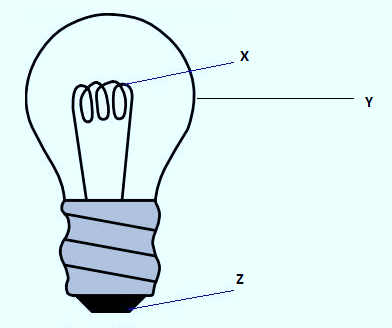(p)X (a)Terminals (q)Y (b)Filaments (r)Z (c)Electric bulb
(a) P -> A, Q ->B, R-> C
(b) P -> B, Q ->C, R-> A
(c) P -> C, Q ->B, R->A
(d) None of these

Question 12
In the below arrangement, the bulb will not glow, if it is connected to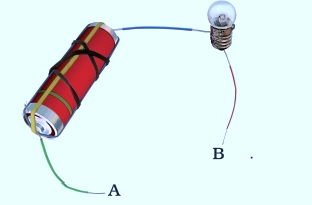(a) copper wire
(b) metal clip
(c) steel spoon
(d) Plastic

Question 13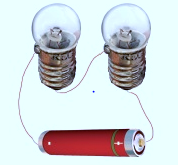Which is of these is true
(a) If the first bulb filament is broken, the second bulb will still glow
(b) If the second bulb filament is broken, the first bulb will still glow
(c) Both the bulb will glow even if one of the bulb filament is broken
(d) Neither of the bulb will glow

Question 14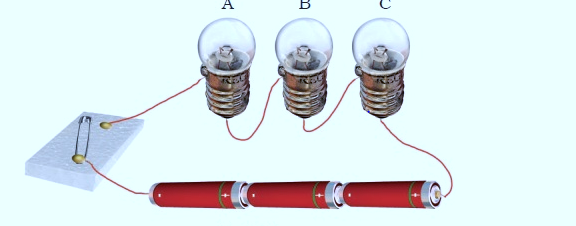In the above diagram, the switch is moved to On position
(a) Bulb A will glow first
(b) Bulb B will glow first
(c) Bulb C will be glow first
(d) All the bulb will glow together

1. (c)
2. (b)
3. (a)
4. (b)
5. (d)
6. (a)
7. (a)
8. (b)
9. (a)
10. (d)
11. (b)
12. (d)
13. (d) As the complete electric circuit is not created
14. (d)

Question 15
1. What kind of cell is used in a wristwatch?
2. From which type of circuit, current can be drawn from the cell?
3. What is solar cell?
4. In a primary cell, the chemical energy is converted to which form of energy?
5. What is an electric circuit?
6. What is dry cell?
7. Name two appliances that work on electricity.

(i) Button cell
(ii) Complete circuit
(iii) A device which converts solar energy into electrical energy.
(iv) Electrical energy.
(v) An electric circuit is a path through which the current flows.
(vi) It is a device which converts chemical energy into electrical energy
(vii) Refrigerator/television/computer/micro oven/ induction cooker

Question 16
Mark ‘True’ or ‘False’ for the following statements & give reason for your answer (a) Electric current can flow through metals.
(b) Instead of metal wires, a jute string can be used to make a circuit.
(c) Electric current can pass through a sheet of Thermocol.

(a) True; Metals are good conductors of electricity. So, an electric current can easily flow through them.
(b) False; Jute string is a bad conductor of electricity which means it does not allow electric current to flow through it. Hence, it cannot be used to make a circuit.
(c) False; Thermocol is a bad conductor of electricity. Hence, electric current cannot pass through it.### Practice Question

Question 1 What is $\frac {1}{2} + \frac {3}{4}$ ?
A)$\frac {5}{4}$
B)$\frac {1}{4}$
C)$1$
D)$\frac {4}{5}$
Question 2 Pinhole camera produces an ?
A)An erect and small image
B)an Inverted and small image
C)An inverted and enlarged image
D)None of the above